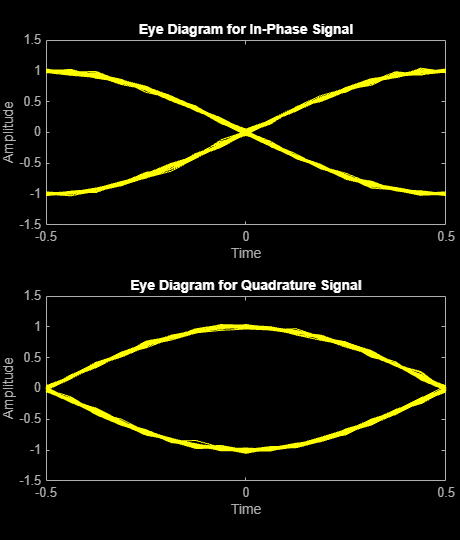# mskmod

Minimum shift keying modulation

## Syntax

```y = mskmod(x,nsamp) y = mskmod(x,nsamp,dataenc) y = mskmod(x,nsamp,dataenc,ini_phase) [y,phaseout] = mskmod(...) ```

## Description

`y = mskmod(x,nsamp)` outputs the complex envelope `y` of the modulation of the message signal `x` using differentially encoded minimum shift keying (MSK) modulation. The elements of `x` must be 0 or 1. `nsamp` denotes the number of samples per symbol in `y` and must be a positive integer. The initial phase of the MSK modulator is 0. If `x` is a matrix with multiple rows and columns, the function treats the columns as independent channels and processes them independently.

`y = mskmod(x,nsamp,dataenc)` specifies the method of encoding data for MSK. `dataenc` can be either `'diff'` for differentially encoded MSK or `'nondiff'` for nondifferentially encoded MSK.

`y = mskmod(x,nsamp,dataenc,ini_phase)` specifies the initial phase of the MSK modulator. `ini_phase` is a row vector whose length is the number of channels in `y` and whose values are integer multiples of `pi/2`. To avoid overriding the default value of `dataenc`, set `dataenc` to `[]`.

`[y,phaseout] = mskmod(...)` returns the final phase of `y`. This is useful for maintaining phase continuity when you are modulating a future bit stream with differentially encoded MSK. `phaseout` has the same dimensions as the `ini_phase` input, and assumes the values `0`, `pi/2`, `pi`, and `3*pi/2`.

## Examples

collapse all

Generate a random binary signal.

`x = randi([0 1],100,1);`

MSK modulate the data.

`y = mskmod(x,8,[],pi/2);`

Pass the signal through an AWGN channel. Display the eye diagram.

```z = awgn(y,30,'measured'); eyediagram(z,16);```## References

 Pasupathy, S., "Minimum Shift Keying: A Spectrally Efficient Modulation". IEEE Communications Magazine, July, 1979, pp. 14–22.

## Version History

Introduced before R2006a